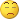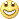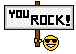# All John Ehlers Indicators... - page 54201591

sabbir50:
Any one please post MTF Fisher or Solar Wind No repaint with alert Indicator ....You can use the one from this post : https://www.mql5.com/en/forum/173574/page360

It is a multi time frame version with alerts in it already298

FDI indicator

Recently I was playing with HE in MATLAB as I'm trying to go more deep into FX data structure and unfortunately i have bad news about FDI indicator from John

in this post i came to the conclusion that using HE for trading is kind of useless as it is unstable - HE was calculated by MATLAB wfbmesti function using 3 different methods

https://www.mql5.com/en/forum/178285/page6

Than I compared this output with John's output which was looking much more stable and smooth and I started to be suspicious - Johns smooths price twice before and after calculation. Well maybe is OK do it after but before I suspected it can destroy the distribution structure.

So I made the experiment. I generated fractional Brownian Motion with known HE value and calculated HE from this as a reference.

Than I smoothed generated series and calculate HE to see if there is an impact of smoothing for HE. so:

% Set parameter H to 0.6 and sample length

H = 0.6; lg = 10000;

% Generate 100 wavelet-based fBm realizations for H = 0.6

% and compute the three estimates for each of them

n = 100; Hest = zeros(n,3);

for i = 1:n

fBm06 = wfbm(H,lg);

Hest(i,:) = wfbmesti(fBm06);

end

mean (Hest)

ans =

0.5927 0.5927 0.5648[/CODE]

Values of HE of generated series are around 0.6 as expected. John uses this formula for smoothing

Smooth = (Price + 2 * Price + 2 * Price + Price ) / 6 ;

so

[CODE]n = 100; Hest2 = zeros(n,3);H = 0.6; lg = 10000;

for i = 1:n

fBm06 = wfbm(H,lg);

for ii = 4:lg

fBm06(ii) = (fBm06(1,ii) + 2 * fBm06(1,ii-1) + 2 * fBm06(1,ii-2) + fBm06(1,ii-3) ) / 6 ;

end

Hest2(i,:) = wfbmesti(fBm06(:,4:end));

end

mean (Hest2)

ans =

0.7013 0.5977 0.8760

so not around 0.6 anymore as it should be !!!! Distribution and structure is affected !!! It looks that only method 2

survived this (second order discrete derivative, wavelet-based).Just wonder if Johns method survive this ???

Beside this from the posts with link above when I removed smoothing from the FDI code and plot 2-FDI (so HE) this value is completely different than HE value generated by MATLAB

So in summary I would not trust this indicator and any other which clones his code !!!

Krzysztof289

Krzysztof

If they are, I do not think that they are on a right track (quite frankly it is too complicated to use and the results are not what one would expect from a good average/filter).

Not the question if something is superior to Ehlers' ma (Ehlers is not good in the field of MAs - some his attempts like the one he named "zero lag ma" are frankly anything but serious) but a comparison to some much better filters immediately comes to mind and then that ma is not going to be so shiny

Check it out, please...Files:201591

Tsar:

Check it out, please...Tsar

Even though they are not the same (code is different and it is obvious that it is written by different persons), calculated values are exactly the same when the parameters are the same (for final result as well as for the "primary" result). So those two are two versions of the same indicator289

Tsar Even though they are not the same (code is different and it is obvious that it is written by different persons), calculated values are exactly the same when the parameters are the same (for final result as well as for the "primary" result). So those two are two versions of the same indicator

Thanks a lot for the clarification201591

Just like a curiosity - here is what John Ehlers called a "zero lag EMA" (tradestation code)

inputs:

Length( 20 ),

GainLimit( 50 ),

Thresh( 1 ), { see article for threshold explanation }

UseThreshold( true ), { if true, then the threshold is used for the

ShowMe dots and crossing alert - see notes below }

DrawMALines( true ), { if true, the Moving Average lines are plotted }

DrawShowMeDots( false ), { if true, ShowMe dots are plotted when a cross

of EC and EMA are detected }

DotOffsetTicks( 10 ), { offset for the ShowMe dots }

DrawPaintBars( false ) ; { draw PaintBars, color depending on EC > EMA }

variables:

ATick( 0 ),

alpha( 0 ),

Gain( 0 ),

BestGain( 0 ),

EC( 0 ),

Error( 0 ),

LeastError( 0 ),

EMA( 0 ),

CrossOver( false ),

CrossUnder( false ) ;

if CurrentBar = 1 then

ATick = MinMove / PriceScale ; { calculate value of a tick }

alpha = 2 / ( Length + 1 ) ;

EMA = alpha * Close + ( 1 - alpha ) * EMA ;

LeastError = 1000000 ;

for Value1 = -GainLimit to GainLimit

begin

Gain = Value1 / 10 ;

EC = alpha *( EMA + Gain * ( Close - EC ) ) + ( 1 - alpha ) * EC ;

Error = Close - EC ;

If AbsValue( Error ) < LeastError then

begin

LeastError = AbsValue( Error ) ;

BestGain = Gain ;

end ;

end ;

EC = alpha * ( EMA + BestGain * ( Close - EC ) ) + ( 1 - alpha ) * EC ;

if DrawMALines then

begin

Plot1( EC, "EC" ) ;

Plot2( EMA, "EMA" ) ;

end ;

It should not be taken serriously since it is one of the cases when John Ehlers was jumping first and only then thinking of what to do (that is the reason why it is not converted to metatrader either)3231

Can it be converted to metatrader nevertheless?201591

Can it be converted to metatrader nevertheless?

Believe me, better not to. That is an artificial way of fitting one value (EMA) to another value (price). I believe that if he could, John Ehlers would revoke that code now, since it is anything but a serious code and average3231